Video Tutorial What is Organic Chemistry?

Organic chemistry is the study of molecules that contain covalently bonded carbon atoms. Carbon is a very useful element in nature because it can form four covalent bonds (group 4 element). This capacity to bond to four other atoms or ‘groups’ gives rise to many different combinations and possible structures involving carbon. As a result, there are millions of organic molecules. Some are naturally occurring (for example sugars, DNA and alcohols) and some are synthesised (made) by chemists.

Quick Notes Organic Chemistry - Introduction

• Empirical formula shows the simplest whole number ratio of elements within a molecule.
• Molecular formula shows the actual number of atoms of each element in a molecule.
• Structural formula shows how the atoms are arranged in a molecule.
• Displayed formula shows a drawing of the arrangement of atoms in a molecule.
• Skeletal formula shows the carbon backbone and functional groups within molecules, no carbon-hydrogen bonds are shown.

Full Notes Organic Chemistry - Introduction

There are several ways of representing organic molecules. Each formula has its own use.

If, for example, we have an organic molecule that contains six carbon atoms and fourteen hydrogen atoms there are different ways we can show the molecule.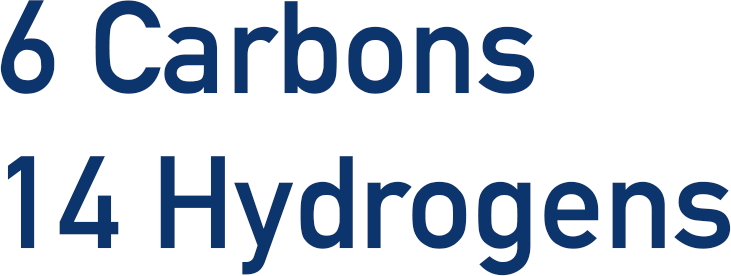Empirical Formula – the simplest, whole number ratio of elements within a compound: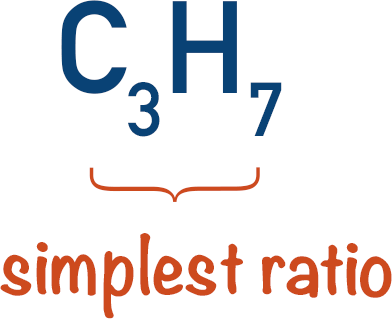Molecular Formula – the actual number of atoms of each element in a molecule: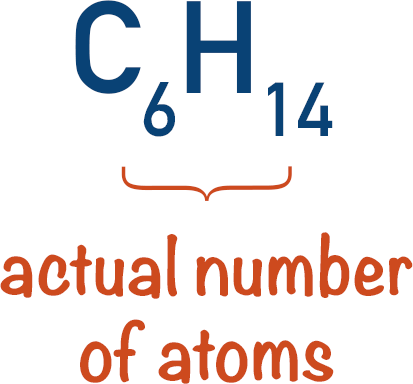Structural Formula – how atoms are arranged in a molecule: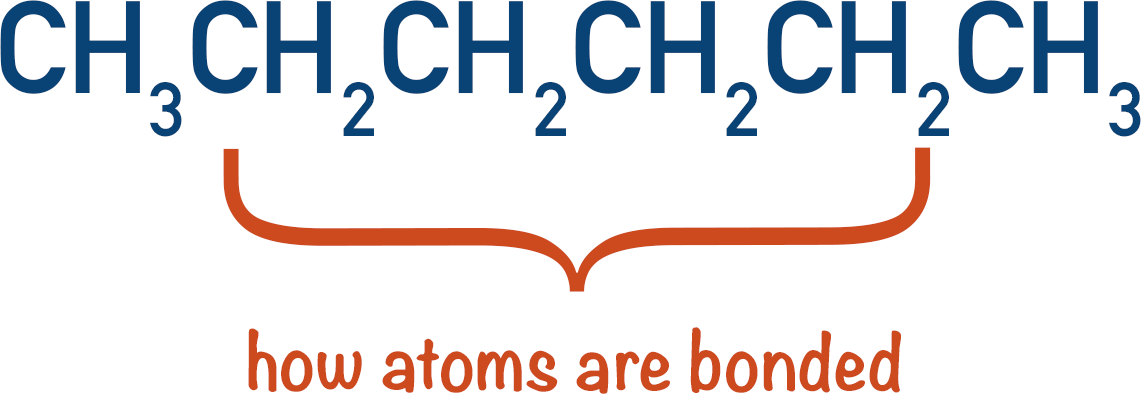Displayed Formula – a drawn out structure, showing the place of atoms in a molecule: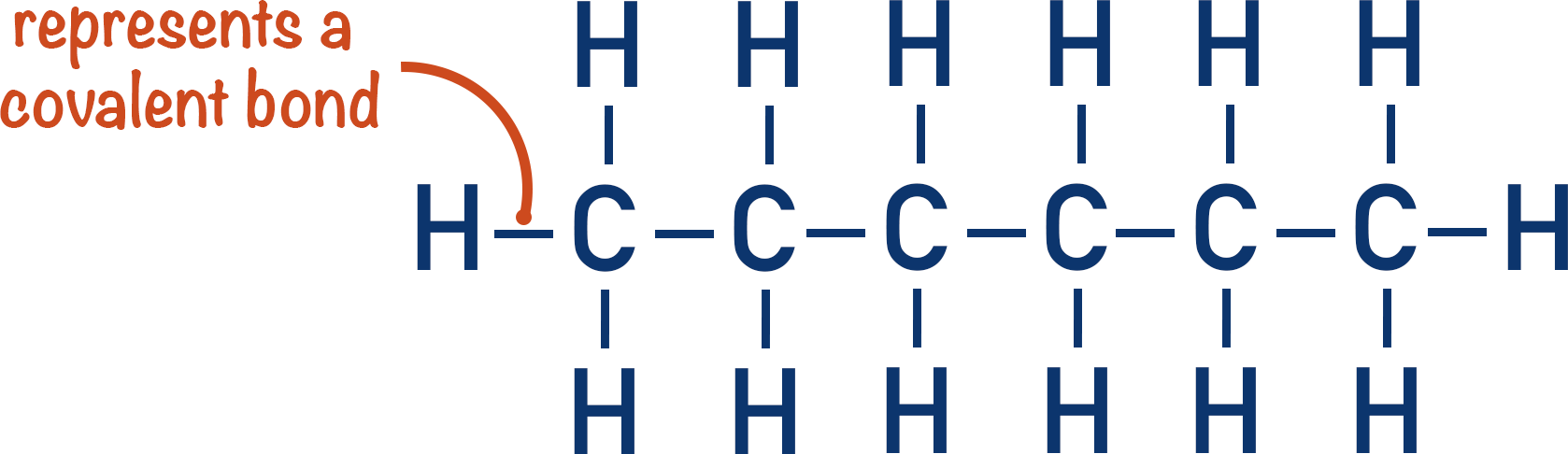Skeletal Formula – simple structure showing only the carbon-carbon bonds and functional groups:Being able to use and understand different formulas is a very important skill in organic chemistry and should not be overlooked!